Function Repository Resource:

# PopovDecomposition

Compute the Popov decomposition of a matrix of univariate polynomials

Contributed by: Daniel Lichtblau
 ResourceFunction["PopovDecomposition"][mat] computes the Popov decomposition of the matrix mat consisting of univariate polynomials. ResourceFunction["PopovDecomposition"][mat,x] gives the Popov decomposition of the matrix mat regarded as univariate polynomials in the variable x.

## Details and Options

The result is given in the form {u,pop} where u is a unimodular matrix, pop is the Popov normal form for mat and u.mat=pop.
The Popov normal form can be characterized as follows. Take the maximal degrees in each column, and permute columns so these are ordered from largest to smallest. Remove any rows of zeros. Now take the matrix of coefficients of maximal degree in each row. This matrix has only nonzero constants on the main diagonal, and zeros everywhere else. Stated differently, after permuting columns to correspond to decreasing maximal degree, the maximal degrees in each row appear on the main diagonal, and these degrees are non-increasing.
The Popov normal form is sometimes referred to as the lattice-reduced form, as it satisfies a minimal degree property analogous to the (approximately) minimal-length behavior of rows of a reduced-integer lattice.

## Examples

### Basic Examples (4)

Compute the Popov decomposition for a 3×3 matrix of quartic polynomials:

 In:=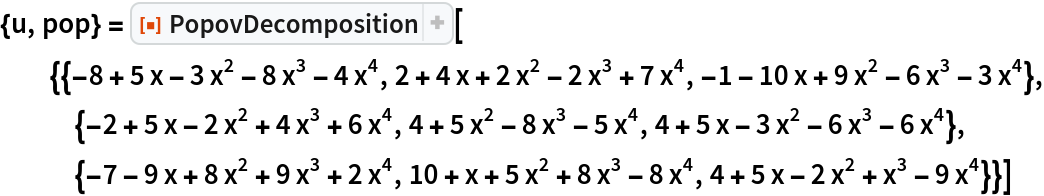Out=Check that the determinant is a constant:

 In:=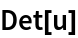Out=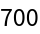Check that the necessary matrix identity is satisfied:

 In:=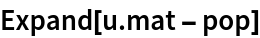Out=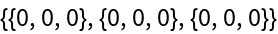Check that the maximal degrees in each row move successively to the right:

 In:=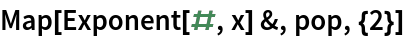Out=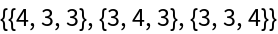### Scope (2)

Create a tool to generate a 3×3 matrix of quartic polynomials:

 In:=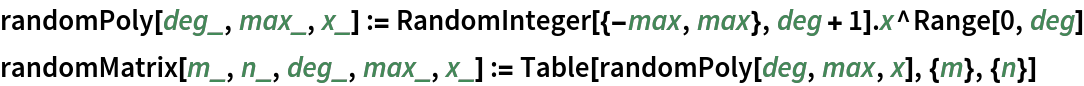If the input matrix does not have full row rank, there will be zero rows at the bottom of the Popov form (as many as the row rank deficiency):

 In:=Out=### Options (3)

Generate a 3×3 matrix of quartic polynomials:

 In:=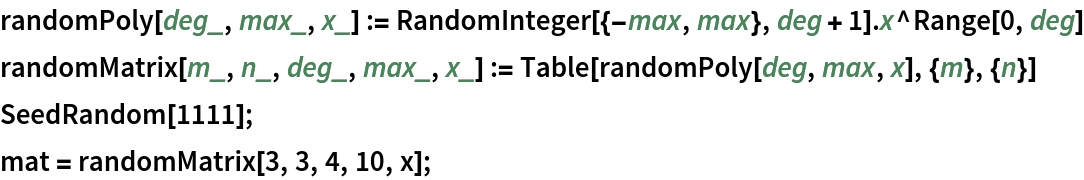Compute the Popov decomposition over the integers modulo a prime:

 In:=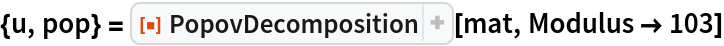Out=Check the necessary matrix identity:

 In:=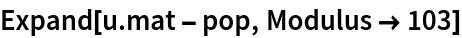Out=## Version History

• 2.0.0 – 05 June 2020
• 1.0.0 – 12 November 2019